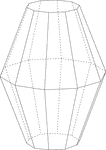Decagonal Bifrustum

Illustration of a decagonal bifrustum created by three parallel planes of decagons with the middle plane…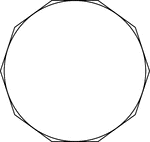Illustration of a decagon circumscribed about a circle. This can also be described as a circle inscribed…Decagon Inscribed In A Circle

Illustration of a decagon inscribed in a circle. This can also be described as a circle circumscribed…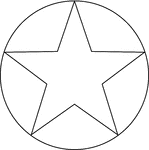Star Inscribed In A Circle

Illustration of a 5-point star inscribed in a circle. This can also be described as a circle circumscribed…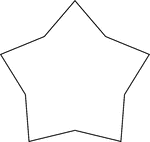Concave Equilateral Decagon

A 5-point star made from a non-regular concave decagon in which all sides are equal in length.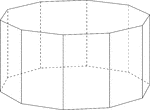Decagonal Prism

Illustration of a decagonal prism with regular decagons for bases and rectangular faces. The hidden…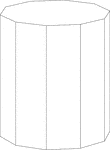Decagonal Prism

Illustration of a right decagonal prism with regular decagons for bases and rectangular faces.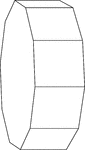Decagonal Prism Resting On Its Side

Illustration of a right decagonal prism with regular decagons for bases and rectangular faces. The prism…Swimming Pool Shaped Like A Decagonal Prism

Illustration of a swimming pool and water hose that is in the shape of a hollow regular decagonal prism…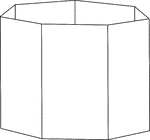Heptagonal/Septagonal Prism

Illustration of a hollow right heptagonal/septagonal prism with regular heptagons/septagons for bases…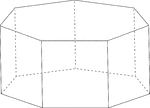Heptagonal/Septagonal Prism

Illustration of a regular right heptagonal/septagonal prism with regular heptagons/septagons for bases…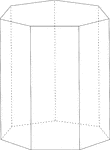Heptagonal/Septagonal Prism

Illustration of a right heptagonal/septagonal prism with regular heptagons/septagons for bases and rectangular…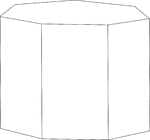Heptagonal/Septagonal Prism

Illustration of a right heptagonal/septagonal prism with regular heptagons/septagons for bases and rectangular…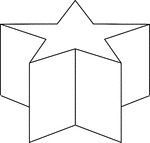Star-Shaped Decagonal Prism

Illustration of a non-regular decagonal prism in the shape of a star. Then ends/bases are made of star-shaped…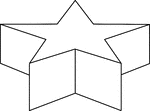Star-Shaped Decagonal Prism

Illustration of a non-regular decagonal prism in the shape of a star. Then ends/bases are made of star-shaped…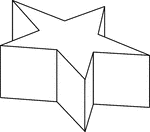Star-Shaped Decagonal Prism

Side view of a non-regular decagonal prism in the shape of a star. Then ends/bases are made of star-shaped…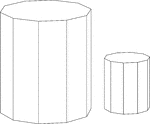Similar Decagonal Prisms

Illustration of 2 similar right decagonal prisms. Both have regular decagons for bases and rectangular…